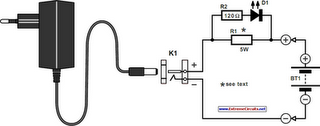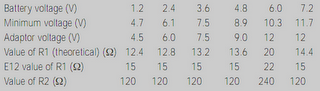# Learning Electronics

Learn to build electronic circuits

# NiCd Battery Charger

The design of the charger is similar to that of many commercially available chargers. The charger consists of a mains adaptor, two resistors and a light-emitting diode (LED). In practical use, this kind of charger is perfectly all right. Resistor R1 serves two functions: it establishes the correct charging current and it drops sufficient voltage to light the diode. This means that the LED lights only when a charging current flows into the battery. The charging current is about 1/4 of the battery capacity, which allows a slight overcharging, and yet the charging cycle is not too long (4–5 hours).

Circuit diagram:NiCd Battery Charger Circuit Diagram

The value of the resistors may be calculated as follows, for which the nominal e.m.f. and the capacity of the battery must be known. Adjust the output of the mains adaptor to 1.17 times the nominal battery voltage plus 3.3 V, which is the potential across R1. Note that the adaptor must be capable of supplying a current of not less than half the battery capacity. The value of R1 in ohms is equal to 3.3 divided by 1/4 of the battery capacity. The value of the resistors for various battery voltages is given in the Table. The battery capacity is taken as 1 Ah.The rating of R1 should be 5 W. If the battery to be charged has a different capacity, the theoretical value of R1 in the table must be divided by the battery capacity. Its actual value is the nearest one in the E12 series. For instance, if a 6 V battery with a nominal capacity of 600 mAh is to be charged, the value of R1 must be 20/0.6 = 33R.